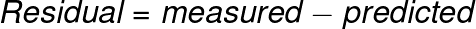# Residual Calculator

Or input your data as csv

Sharing helps us build more free tools

The residual is the difference between the predicted and actual values in a regression model.Sometimes it is also useful to know the standard residuals, squared residuals, standard deviation of the residuals, or the residual sum of squares.

## What is the Residual Used For In Regression

Residuals are often used to see whether a linear regression model has violated any of the assumptions of linear regression.

The easiest way to look at residuals is with a residual plot. That’s just a scatter plot where the dependent variable is on the x axis and the residuals are plotted on the y axis.

If your residuals do not look like they have a constant various variance overall values of x then you may have violated one of the assumptions of linear regression. Similarly,  if the residuals are not normally distributed over all values of x then you may have violated another assumption of linear regression.

Residual plots make it really make it easier to see if you have violated some of the linear regression model assumptions.

## How To Calculate the Residual For a Regression Model

The residual is just the difference between the measured value and the predicted value.

We can get the residuals of a linear regression model by calculating the difference between each measurement and the corresponding predicted value.